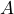# A. Chiarini & M. Nitzschner: Disconnection and entropic repulsion for the harmonic crystal with random conductances

Abstract: We study level-set percolation of the discrete Gaussian free field on the Euclidean lattice in three and more dimensions, equipped with uniformly elliptic random conductances. We prove that this percolation model undergoes a non-trivial phase transition at a deterministic level. For a compact setin, we study the disconnection event that the level-set of the field below a given level disconnects the discrete blow-up offrom the boundary of an enclosing box, in a strongly percolative regime. We present quenched asymptotic upper and lower bounds on this probability in terms of the homogenized capacity of. Moreover, we investigate the behavior of the field conditioned on the disconnection event.

The first half of the talk will focus on introducing the model and describing the relevant critical parameters that capture its percolative behavior. We put the model into context, and present the main bounds for the disconnection probability. In the second half of the talk we will present an outline of the proof of these bounds, and discuss the role of novel “solidification estimates” for random walks, which are similar in nature to the corresponding estimates for Brownian motion.

## Video